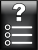<< Previous

JLPT 5 Kanji: Numbers

When counting objects using the counter "つ" then the Kun-Yomi is used. When counting in general the On-Yomi is used.

1) 一 (# 2 of 2,500)

Examples
KunOn

(ひと.つ)
one
(いち)
one

2) 二 (# 9 of 2,500)

Examples
KunOn

(ふた.つ)
two
(に)
two

3) 三 (# 14 of 2,500)

Meaning: three

Examples
KunOn

(みっ.つ)
three
(さん)
three

4) 四 (# 47 of 2,500)

Meaning: four

Examples
KunOn

(よっ.つ)
four
(よん)
four

5) 五 (# 31 of 2,500)

Meaning: five

Examples
KunOn

(いつ.つ)
five
(ご)
five

6) 六 (# 93 of 2,500)

Meaning: six

Examples
KunOn

(むっ.つ)
six
(ろく)
six

7) 七 (# 115 of 2,500)

Meaning: seven

Examples
KunOn

(なな.つ)
seven
(なな)
seven

8) 八 (# 92 of 2,500)

Meaning: eight, eight radical (no. 12)

Examples
KunOn

(やっ.つ)
eight
(はち)
eight

9) 九 (# 55 of 2,500)

Meaning: nine

Examples
KunOn

(ここの.つ)
Nine
(きゅう)
nine

10) 十 (# 8 of 2,500)

Meaning: ten

Examples
KunOn
(とお)
10, ten
(じゅう)
ten

11) 百 (# 163 of 2,500)

Meaning: hundred

Examples
KunOn
NONE
(ひゃく)
hundred

12) 千 (# 195 of 2,500)

Meaning: thousand

Examples
KunOn
NONE
(せん)
thousand

13) 万 (# 375 of 2,500)

Meaning: ten thousand, 10,000

Examples
KunOn
NONE
(まん)
10, 000

14) 半 (# 224 of 2,500)

Meaning: half, middle, odd number, semi-, part-

Examples
KunOn

(なか.ば)
middle, half,
semi, halfway,
partly
(はん)
half

Quizzes
Now it's time to start the Quizzes!! First take the meaning quiz:

(click)Numbers

Once you have a space in your brain for the Kanji and it's meaning it will be easier to add Kun-Yomi and On-Yomi words:

(click)Kun-Yomi

(click)On-Yomi